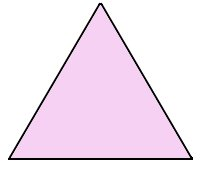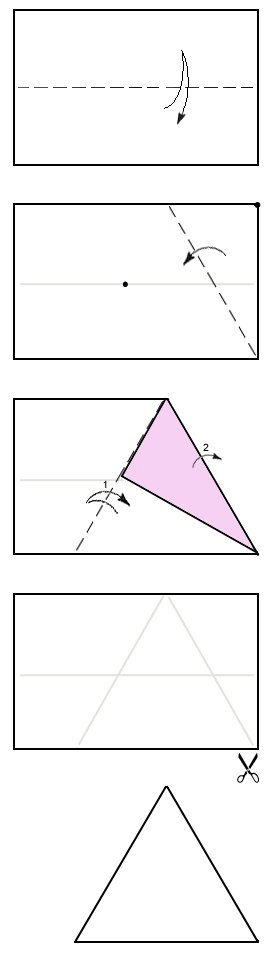# Triangle from a RectangleSome origami models start with an equilateral triangle instead of a square sheet of paper. In this case, you need to make the triangular sheets of paper before you start. This page will show you how to get a triangle from a rectangular sheet of paper.

If you are starting with a square sheet of paper, or a rectangle that is not quite long enough, then click here or here.#### Make a Triangle from a Rectangle

1. Fold and unfold the rectangular sheet of paper in half lengthwise.

2. Fold the top-right corner of the paper down so the corner meets the crease made in step 1 (join the dots). The crease made should intersect with the bottom-right corner.

3. Fold left side of the paper over the flap made in step 2. Use the raw edge of the flap as a guide to position this fold.

4. Unfold and cut along the creases to get an equilateral triangle. Easy.